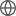Old Web
English
# The HETDEX Survey: The Ly$\alpha$ Escape Fraction from 3D-HST Emission Line Galaxies at $z \sim 2$
We measure the Ly$\alpha$ escape fraction of 935 [OIII]-emitting galaxies between $1.9 < z < 2.35$ by comparing stacked spectra from the Hubble Space Telescope/WFC3's near-IR grism to corresponding stacks from the Hobby Eberly Telescope Dark Energy Experiment's Internal Data Release 2. By measuring the stacks' H$\beta$ to Ly$\alpha$ ratios, we determine the Ly$\alpha$ escape fraction as a function of stellar mass, star formation rate, internal reddening, size, and [OIII]/H$\beta$ ratio. We show that the escape fraction of Ly$\alpha$ correlates with a number of parameters, such as galaxy size, star formation rate, and nebular excitation. However, we also demonstrate that most of these relations are indirect, and the primary variables that control the escape of Ly$\alpha$ are likely stellar mass and internal extinction. Overall, the escape of Ly$\alpha$ declines from $\gtrsim 18\%$ in galaxies with $\log M/M_{\odot} \lesssim 9$ to $\lesssim 1\%$ for systems with $\log M/M_{\odot} \gtrsim 10$, with the sample's mean escape fraction being $6.0^{+0.6\%}_{-0.5\%}$.